## Motivation: Predicting a Noisy Time Series

To illustrate the value of probabilistic forecasts, let’s take a look at some different approaches of predicting a time series with a variable noise component:Variable noise component

The time series on top represents the quantity that we want to predict. It is an artificially created sine series with a variable gaussian noise component. What’s variable is the intensity of the noise, which itself is also described by a sine curve (although with a different frequency from the other one) as shown in pink. We want to predict the blue validation time series given the historical record in black, and given the knowledge of the noise intensity both in the past and the future. Please note that, while this specific time series is artificial, processes that create varying noise intensities are found in many real-world scenarios (think of marketing budgets for instance).

## Attempt 1: Naive Model

One of the simplest ways to create a forecast would be to use one of the naive baseline models of Darts, namely the `NaiveSeasonal` model. This model accepts a seasonal period as a parameter, and at prediction time simply repeats the last observed value that was at the same point in the seasonal cycle.

naive_model = NaiveSeasonal(seasonal_period)
naive_model.fit(target_train)
naive_model.predict(n)Naive Model

As you can see, this is not a very good result. The problem is that the model also predicts the same noise as it saw before, even though the noise is by definition not predictable.

## Attempt 2: Autoregressive Model

Our previous attempt was unsuccessful because our model did not separate signal from noise. To address this issue, let’s try a more sophisticated forecasting model: A simple autoregressive model. For this, we can use the ARIMA model of Darts with a non-zero value for only the first parameter.

ar_model = ARIMA(seasonal_period, 0, 0)
ar_model.fit(target_train)
ar_model.predict(n)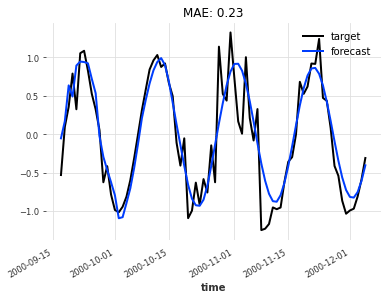Autoregressive Model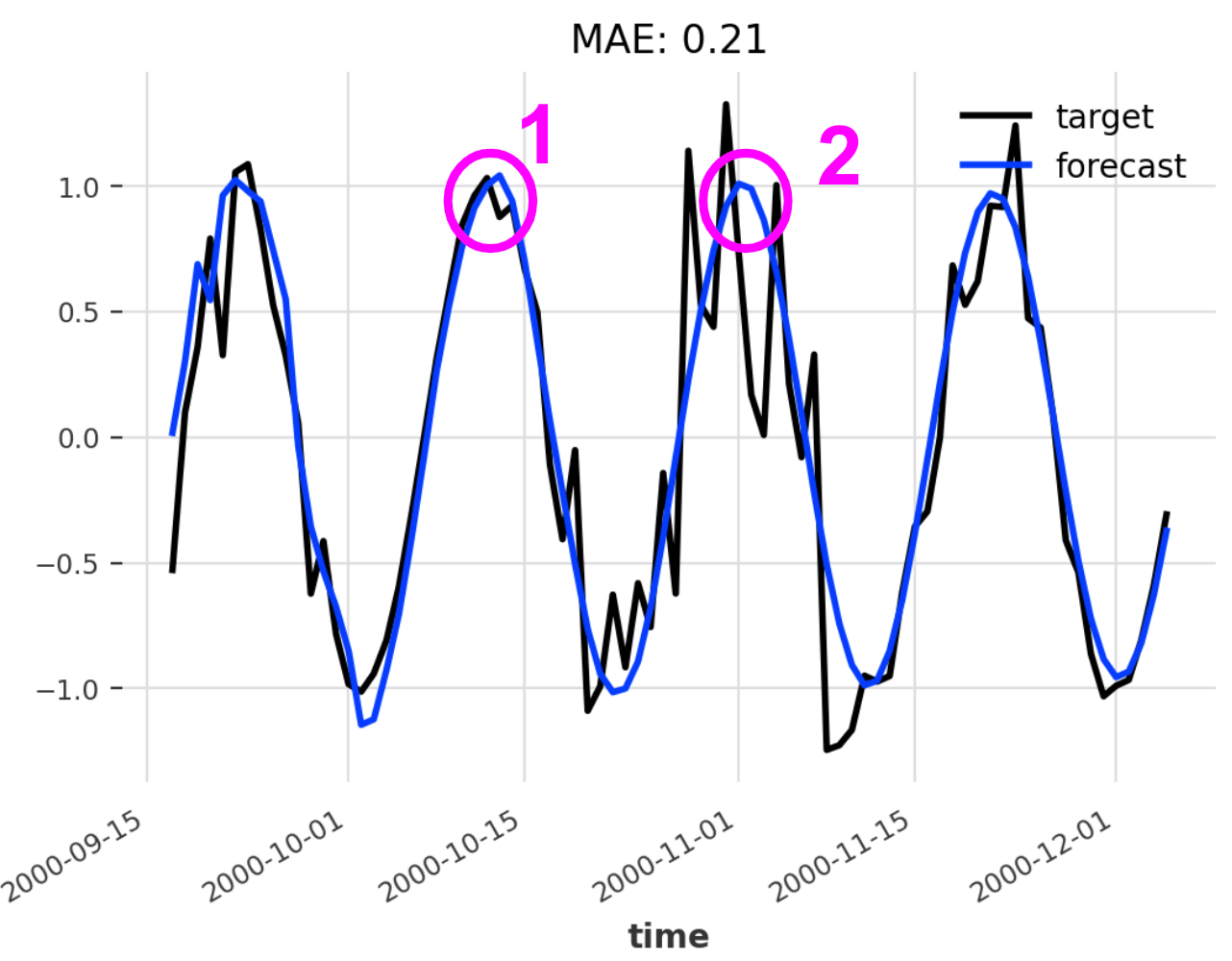Two point predictions

Prediction 1 could be considered a ‘safe’ forecast because the noise component is weak at this point in time. In other words, we can be fairly certain that the ground truth value is close to our forecast. However, if we look at prediction 2, it is much more likely that it will deviate from the ground truth since the noise component is very strong here. Of course, this is obvious to us since we see both the forecast in blue and the ground truth in black. But in a real-world scenario, you would only see the blue line, which gives no indication about the fact that one forecast is much ‘safer’ than the other.

## Attempt 3: Probabilistic Model

We want a prediction that doesn’t just provide us the value that, according to the model, is most likely going to be close to the ground truth, but we also want a measure for how certain we can be about that value. To do this we will use one of Darts’ deep learning models, the TCN, in its probabilistic form. To do that we need to make sure we specify the `likelihood` parameter when instantiating the model. And to produce a stochastic forecast, we need to set the `num_samples` parameter to an integer higher than 1. What these two things do exactly will be explained in the next sections.

prob_model = TCNModel(
input_chunk_length=seasonal_period*2,
output_chunk_length=seasonal_period,
dropout=0,
likelihood=GaussianLikelihood()
)
prob_model.fit(target_train, past_covariates=noise_intensity)
pred = prob_model.predict(n, num_samples=100)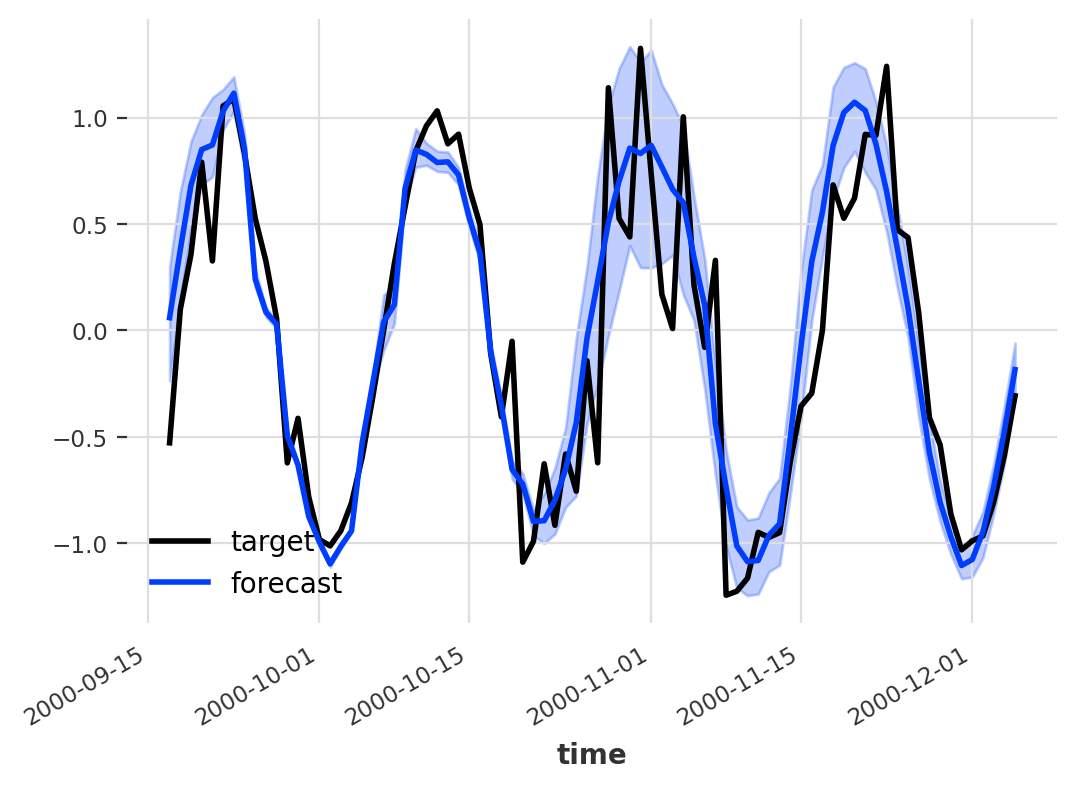Probabilistic Model

Now we’re talking. In addition to one single value per time step which represents the most likely value according to the model (dark blue line), a probabilistic forecast in Darts also provides us with a measure of uncertainty in the form of confidence intervals (light blue area). The wider the confidence interval, the more uncertain the model is about its prediction. But what is happening behind the scenes when we create a probabilistic forecast, and how do we get these confidence intervals? To find out, please read on.

## Deterministic vs. Stochastic Forecasts

deterministic model, once trained, can be seen as a function that maps a given input series (and optionally covariates) to a deterministic forecast. A deterministic forecast in Darts is a `TimeSeries` instance with shape `(length, num_components, 1)` where `length` corresponds to the number of predicted time steps, and `num_components` represents the dimensionality of the time series at one specific point in time (which is equal to 1 for a univariate time series). In other words, given a point in time and given a time series component, there is exactly one value that fully describes the forecast at that point.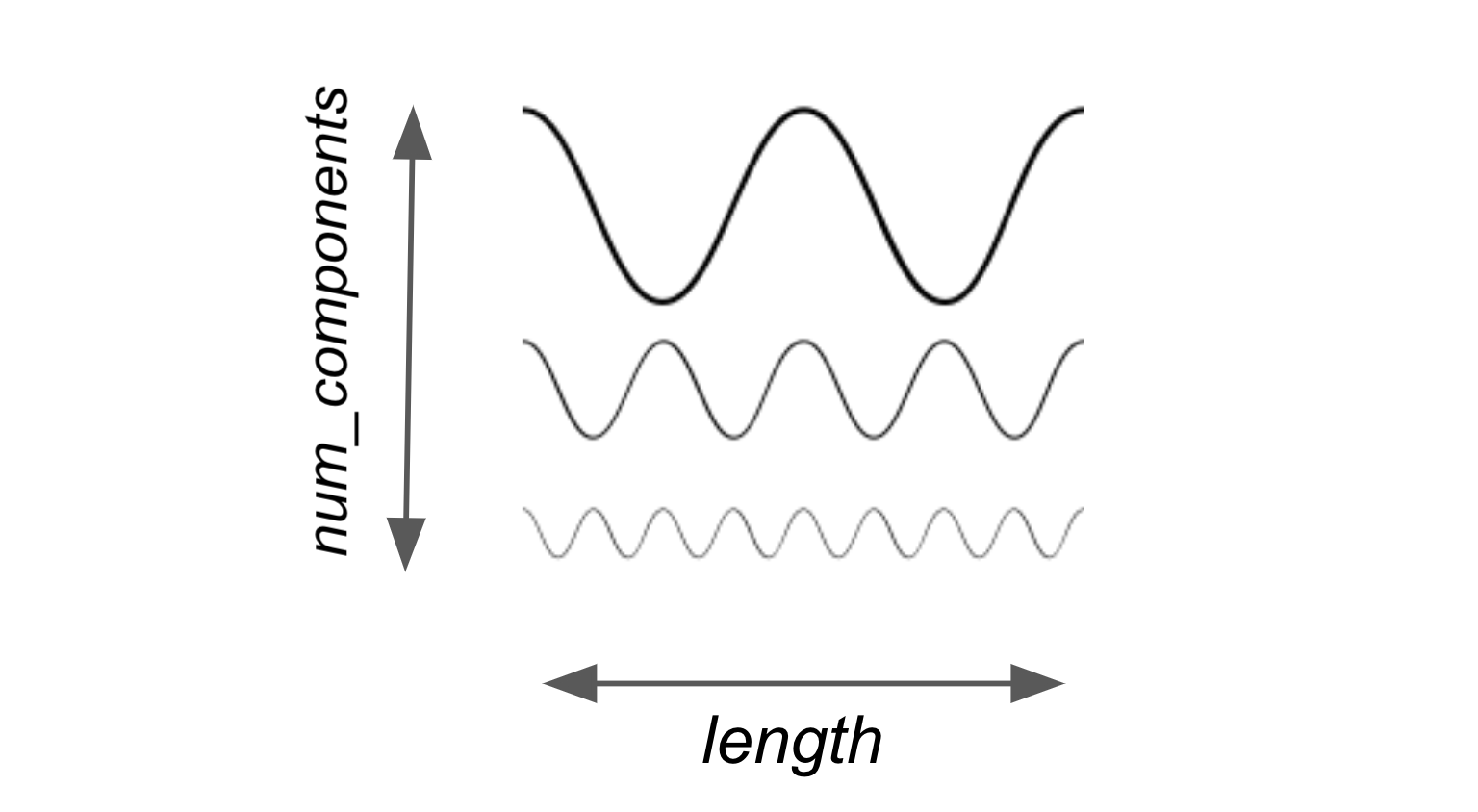Deterministic time series in Darts

A trained probabilistic model, rather than a deterministic function mapping input to output, can be seen as a mapping of an input series to a random variable. However, since in general there is no guarantee of a nice analytic description of this random variable, the act of predicting will return samples from this random variable as a forecast. A probabilistic forecast is thus a `TimeSeries` instance with dimensionality `(length, num_components, num_samples)`. The first two dimensions have the same meaning as in the deterministic case. The last dimension represents the different samples that are produced by the probabilistic model.Stochastic time series in Darts

## Interpreting Stochastic Forecasts

Given a set of samples of the random variable defined by a probabilistic model together with an input series, we can compute statistics that help us estimate the ranges of values that we can expect a value in the future to assume. The most important statistic that is used in Darts to establish these ranges is the quantile.

pred = prob_model.predict(n, num_samples=100)pred.quantile_timeseries(0.05).plot(color=’cornflowerblue’) pred.quantile_timeseries(0.95).plot(color=’lightsteelblue’)Plot of two quantile time series generated form a probabilistic forecast

pred.quantile_timeseries(0.5).plot()Plot of the median quantile of a probabilistic forecast

pred.plot(low_quantile=0.05, high_quantile=0.95, color=’blue’)Probabilistic forecast plot showing median and confidence interval

## ANNs and Likelihood Models

Up until now, we haven’t gone into the details of how exactly a probabilistic model in Darts captures stochasticity. That was done on purpose since we allow different models to have various mechanisms for this. `ARIMA` and `ExponentialSmoothing` allow sampling by running simulations of their state-space models with randomly sampled errors. Our deep learning-based forecasting models, on the other hand, model probability distributions a bit more explicitly using the Darts `Likelihood` class.

1. It defines how the loss is computed as a function of the distribution parameters and an actual observation. This boils down to taking a numerically stable negative log likelihood of the given distribution of the observation.
2. It provides a way to sample predictions from the distribution defined by the parameters predicted by the model and the probability distribution.

## Real-World Example

To see how you can use Darts to create probabilistic forecasts on real-world datasets, please check out our example notebook.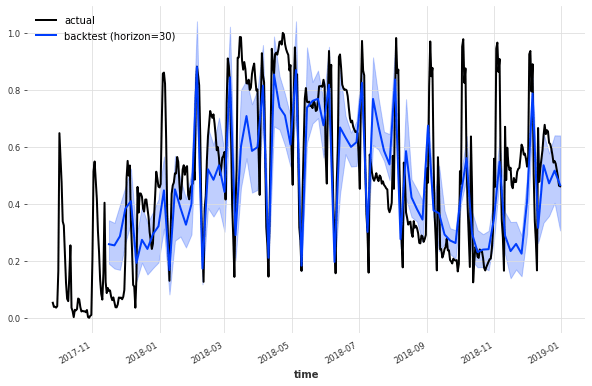Probabilistic TCN forecast of energy production time series

## Conclusion

Given the inherent uncertainty of most quantities humans are interested in predicting, it is crucial to quantify this uncertainty in an informed way. Darts provides the tools to do this in the form of probabilistic forecasting models. The idea behind these models is to represent a random variable parametrized by the input series, where the distribution of this random variable (once the model is trained) should approximate the distribution of the real-time series. Then, this random variable is represented as Monte Carlo samples to create what we call a probabilistic forecast. This forecast is subsequently used to compute statistics that help us quantify uncertainty in our forecast, namely quantiles and confidence intervals.